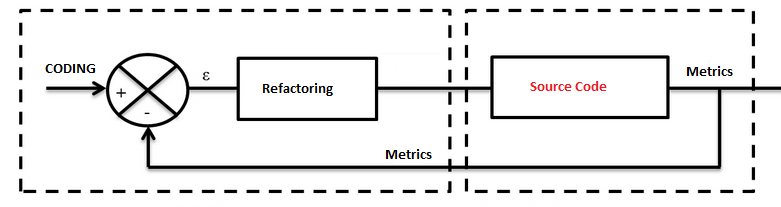The readability of source code has a direct impact on how well a developer comprehends a software system. Code maintainability refers to how easily that software system can be changed to add new features, modify existing features, fix bugs, or improve performance.

Many coding techniques exist to improve the readability and the maintainability. However it’s better to be assisted by some metrics to help refactor the code and keep it clean. We can regulate our code base like an industrial process:Halstead complexity measures are software metrics introduced by Maurice Howard Halstead in 1977 as part of his treatise on establishing an empirical science of software development.

Halstead’s goal was to identify measurable properties of software, and the relations between them.  Thus his metrics are actually not just complexity metrics.

For a given problem, Let:

•$\,\eta_1$ = the number of distinct operators
•$\,\eta_2$ = the number of distinct operands
•$\,N_1$ = the total number of operators
•$\,N_2$ = the total number of operands

From these numbers, several measures can be calculated:

• Program vocabulary:$\eta = \eta_1 + \eta_2 \,$
• Program length:$N = N_1 + N_2 \,$
• Calculated program length:$\hat{N} = \eta_1 \log_2 \eta_1 + \eta_2 \log_2 \eta_2$
• Volume:$V = N \times \log_2 \eta$
• Difficulty :$D = { \eta_1 \over 2 } \times { N_2 \over \eta_2 }$
• Effort:$E = D \times V$

The difficulty measure is related to the difficulty of the program to write or understand, e.g. when doing code review.

The effort measure translates into actual coding time using the following relation,

• Time required to program:$T = {E \over 18}$ seconds

Halstead’s delivered bugs (B) is an estimate for the number of errors in the implementation.

• Number of delivered bugs :$B = {E^{2 \over 3} \over 3000}$ or, more recently,$B = {V \over 3000}$ is accepted

The Halstead complexity measures provide insight into the readability of the code. These count the operators and operands to determine volume, difficulty, and effort. Often, these can indicate how difficult it will be for someone to understand the code.

These metics could be used to improve the readability of the code base and it’s better to refactor when their values are more than the accepted ones to keep your code readable. If you have a C/C++ code base you can use CppDepend to calculate them.

Technical debt

Here’s a definition from this interesting article:

Just like a financial debt, the technical debt incurs interest payments. These are paid in the form of extra effort required to maintain and enhance the software which has either decayed or is built on a shaky foundation. Most Agilists recommend repaying the technical debt as early as possible. However, most Agile teams fail to monetize the technical debt, which can give valuable insights.

• Debt(in man days) = {cost_to_fix_duplications + cost_to_fix_violations + cost_to_comment_public_API + cost_to_fix_uncovered_complexity + cost_to_bring_complexity_below_threshold + cost_to_cut_cycles_at_package_level}

There is a default cost in hour associated with each of the above violation. For example

• cost_to_fix_duplications = {cost_to_fix_one_block * duplicated_block}

Now, as per the defaults cost_to_fix_one_block = 2 hours. Assuming that the average developer cost is $500 per day and there are 8 hours to a day then to fix one such block$125 would be spent. Likewise, monetary analysis can be done for each violation to finally arrive at the total technical debt.

Having more technical debt means that it will become more difficult to continue to develop a system – you either need to cope with the technical debt and allocate more and more time for what would otherwise be simple tasks, or you need to invest resources (time and money) into reducing technical debt by refactoring the code, improving the tests, and so on. Using the Technical debt from the beginning  for continuous improvement  is a good idea to keep the code maintanable. For C/C++ code base you can use the C/C++ Sonar Plugin based on Clang to calculate the technical debt.

Summary

Keep the code base readable and maintainable is not an easy task and using some metrics could help to facilitate this task and give a relevant indicators on where we have to improve our code. Many measures exist and you can choose the ones you consider relevant. But never blindly move forward,  your code will be quickly a labyrinthine system.